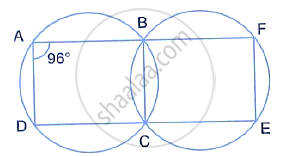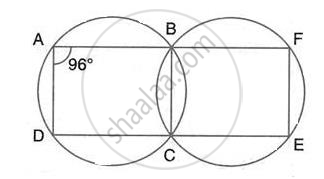Share

In the Following Figure, (I) If ∠Bad = 96°, Find ∠Bcd and ∠Bfe. (Ii) Prove that Ad is Parallel to Fe. - ICSE Class 10 - Mathematics

Question

In the following figure,
(i) If ∠BAD = 96°, find ∠BCD and ∠BFE.
(ii) Prove that AD is parallel to FE.Solution(i) ABCD is a cyclic quadrilateral
∴ ∠BAD + ∠BCD = 180°
(pair of opposite angles in a cyclic quadrilateral are supplementary)
⇒ ∠BCD = 180° - 96° = 84°
∴ ∠BCE = 180° - 84° = 96°
Similarly, BCEF is a cyclic quadrilateral
∴ ∠BCE + ∠BFE = 180°
(pair of opposite angles in a cyclic quadrilateral are supplementary)
∴ ∠BFE = 180° - 96° = 84°

(ii) Now, ∠BAD + ∠BFE = 96 °+ 84° = 180°

But these two are interior angles on the same side

of a pair of lines AD and FE
∴ AD || FE

Is there an error in this question or solution?
Solution In the Following Figure, (I) If ∠Bad = 96°, Find ∠Bcd and ∠Bfe. (Ii) Prove that Ad is Parallel to Fe. Concept: Cyclic Properties.
S